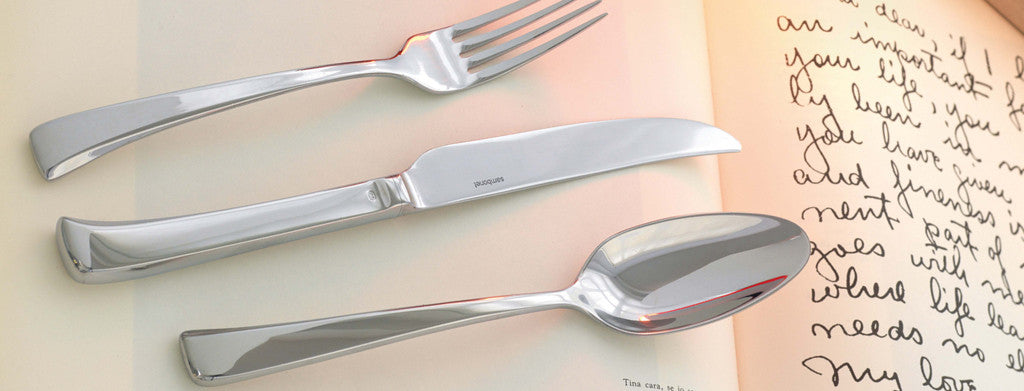Shopping Cart

### Refine

View all

#### Price# Cutlery£3.40 each (ex VAT)£2.52 each (ex VAT)£3.95 each (ex VAT)£3.29 each (ex VAT)£2.52 each (ex VAT)£3.98 each (ex VAT)£5.48 each (ex VAT)£1.71 each (ex VAT)£5.59 each (ex VAT)£3.40 each (ex VAT)£5.59 each (ex VAT)£3.05 each (ex VAT)£6.15 each (ex VAT)£5.48 each (ex VAT)£3.46 each (ex VAT)£3.95 each (ex VAT)£7.57 each (ex VAT)£5.48 each (ex VAT)£1.98 each (ex VAT)£4.89 each (ex VAT)£3.95 each (ex VAT)£3.95 each (ex VAT)£6.15 each (ex VAT)£1.43 each (ex VAT)£6.26 each (ex VAT)£3.89 each (ex VAT)£4.89 each (ex VAT)£4.30 each (ex VAT)£3.05 each (ex VAT)£7.57 each (ex VAT)£2.99 each (ex VAT)£4.98 each (ex VAT)£3.95 each (ex VAT)£6.15 each (ex VAT)£6.76 each (ex VAT)£5.48 each (ex VAT)£3.46 each (ex VAT)£1.71 each (ex VAT)£3.98 each (ex VAT)£3.40 each (ex VAT)£2.63 each (ex VAT)£5.07 each (ex VAT)£6.61 each (ex VAT)£5.80 each (ex VAT)£7.74 each (ex VAT)£7.74 each (ex VAT)£7.74 each (ex VAT)£6.44 each (ex VAT)